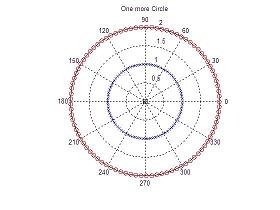# Examples: MATLAB programming - Script Files -

 In this example, we are going to program the plotting of two concentric circles and mark the center point with a black square. We use polar coordinates in this case (for a variation). We can open a new edit window and type the following program (script). As already mentioned, lines starting with a '%' sign are comments, and are ignored by MATLAB but are very useful to the viewer.

% The initial instructions clear the screen, all
% of the variables, and close any figure
clc; clear; close all

% CIRCLE - A script file to draw a pretty circle
% We first generate a 90-element vector to be used as an angle
angle = linspace(0, 2*pi, 90);

% Then, we create another 90-element vector containing only value 2
r2 = linspace(2, 2, 90);

% Next, we plot a red circle using the 'polar' function
polar(angle, r2, 'ro')
title('One more Circle')

% We avoid deletion of the figure
hold on

% Now, we create another 90-element vector for radius 1
r1 = linspace(1, 1, 90);
polar(angle, r1, 'bx')

% Finaly, we mark the center with a black square
polar(0, 0, 'ks')

We save the script and run it with the 'run' icon (within the Editor):Or we can run it from the Command Window by typing the name of the script.

We now get the concentric circles:From 'Matlab Programming' to home

From 'Matlab Programming' to 'Matlab Examples'